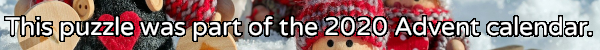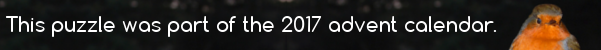mscroggs.co.uk
mscroggs.co.uksubscribe

# Puzzles

## 2 DecemberCarol draws a square with area 62. She then draws the smallest possible circle that this square is contained inside. Next, she draws the smallest possible square that her circle is contained inside. What is the area of her second square?

## 19 DecemberThe diagram below shows three squares and five circles. The four smaller circles are all the same size, and the red square's vertices are the centres of these circles.
The area of the blue square is 14 units. What is the area of the red square?

## Is it equilateral?

In the diagram below, $$ABDC$$ is a square. Angles $$ACE$$ and $$BDE$$ are both 75°.
Is triangle $$ABE$$ equilateral? Why/why not?

## 16 DecemberThere are 204 squares (of any size) in an 8×8 grid of squares. Today's number is the number of rectangles (of any size) in a 2×19 grid of squares

## 14 DecemberThere are 204 squares (of any size) in an 8×8 grid of squares. Today's number is the number of squares in a 13×13 grid of squares

## Squared circle

Each side of a square has a circle drawn on it as diameter. The square is also inscribed in a fifth circle as shown.
Find the ratio of the total area of the shaded crescents to the area of the square.

## Square deal

This unit square is divided into four regions by a diagonal and a line that connects a vertex to the midpoint of an opposite side. What are the areas of the four regions?

## Light work

"I don't know if you are fond of puzzles, or not. If you are, try this. ... A gentleman (a nobleman let us say, to make it more interesting) had a sitting-room with only one window in it—a square window, 3 feet high and 3 feet wide. Now he had weak eyes, and the window gave too much light, so (don't you like 'so' in a story?) he sent for the builder, and told him to alter it, so as only to give half the light. Only, he was to keep it square—he was to keep it 3 feet high—and he was to keep it 3 feet wide. How did he do it? Remember, he wasn't allowed to use curtains, or shutters, or coloured glass, or anything of that sort."

## Archive

Show me a random puzzle
▼ show ▼